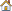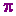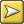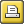﻿ compute new column of dataController: Compute ()The point of it…

This function brings up a calculator, where you can choose functions to calculate values which interest you. For example, a word list routinely provides the frequency of each type, and that frequency as a percentage of the overall text tokens. You might want to insert a further column showing the frequency as a percentage of the number of word types, or a column showing the frequency as a percentage of the number of text files from which the word list was created.This word-list has a column which computes the cumulative scores (running total of the % column).

How to do it

Just press Compute | New Column and create your own formula. You'll see standard calculator buttons with the numbers 0 to 9, decimal point, brackets, 4 basic functions. To the right there's a list of standard mathematical functions to use (pi, square root etc.): to access these, double-click on them. Below that you will see access to your own data in the current list, listing any number-based column-headings. You can drag or double-click them too.Absolute and Relative

Your own data can be accessed in two ways. A relative access (the default) means that as in a spreadsheet you want the new column to access data from another column but in the same row. Absolute access means accessing a fixed column and row.

Examples

 you type Result -- for each row in your data, the new column will contain: Rel(2) ÷ 5 the data from column 2 of the same row, divided by 5 RelC(2) the data from column 2 of the same row, added to a running total Rel(3) + (Rel(2) ÷ 5) the data from column 2 of the same row, divided by 5, added to the data from column 3 of the same row Abs(2;1) ÷ 5 the data from column 2 of row 1, divided by 5. (This example is just to illustrate; it would be silly as it would give the exact same result in every row.) Rel(2) ÷ Abs(2;1) × 100 the data from column 2 of the same row divided by column 2 of row 1 and multiplied by 100. This would give column 3 as a percentage of the top result in column 2. For the first row it'd give 100%, but as the frequencies declined so would their percentage of the most frequent item.

You can format (or even delete) any variables computed in this way: see layout.